# Grade 7 Math STAAR Review Activities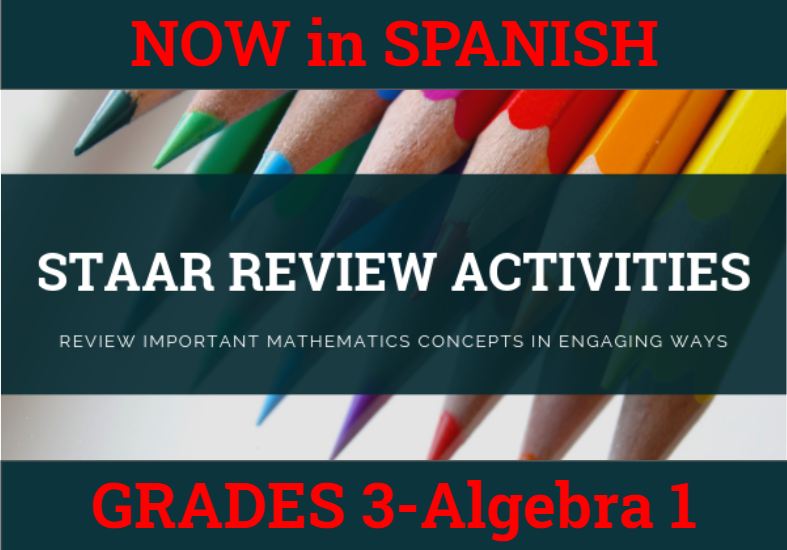How might we purposefully and effectively review important mathematics concepts for the Grade 7 Math STAAR assessment? The ESC-18 Math Team has created a variety of activities where students practice and apply important grade-level TEKS aligned topics to cement their learning.

### Please See Our Pricing and Instructions to Purchase our STAAR Activities

 7.3A, 7.3B Operations with Rational Numbers Task Cards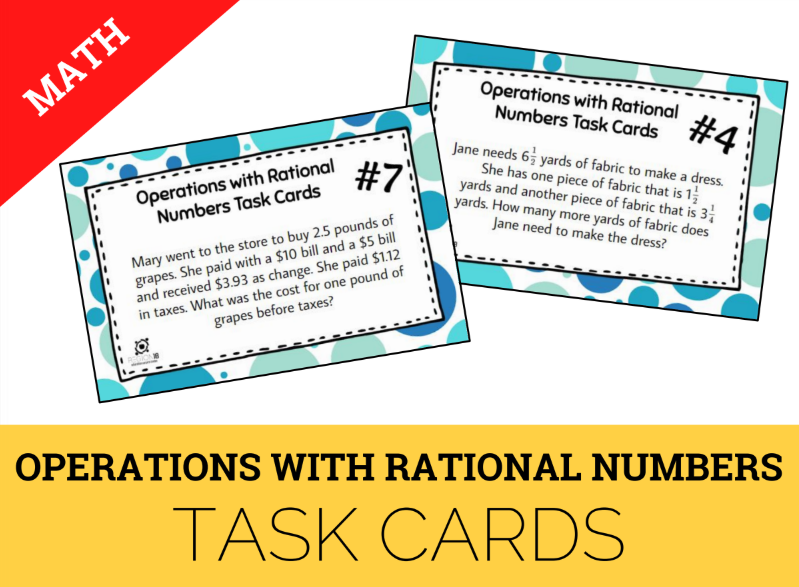Solve problems using addition, subtraction, multiplication, and division of rational numbers. 7.4A Constant Rate of Change Puzzles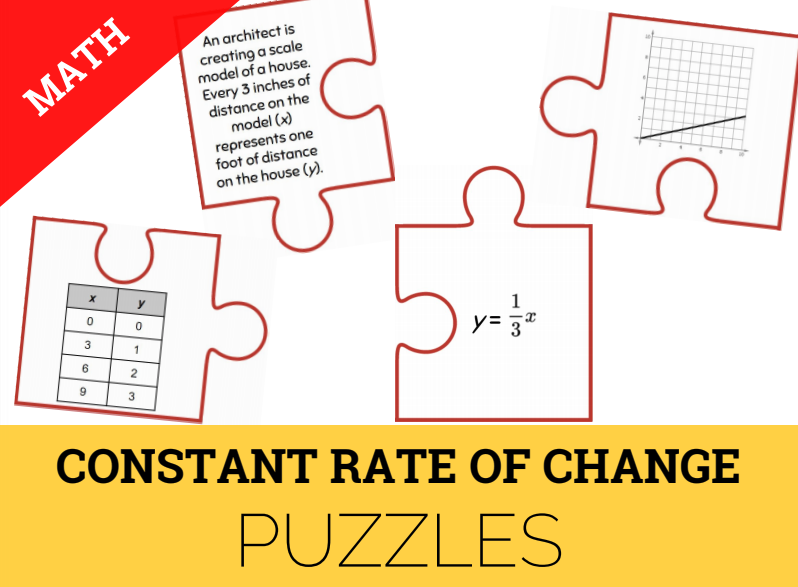Represent constant rates of change in mathematical and real-world problems given pictorial, tabular, verbal, numeric, graphical, and algebraic representations. 7.4D Ratio, Rate, & Percent Multi-Step Problems Scavenger Hunt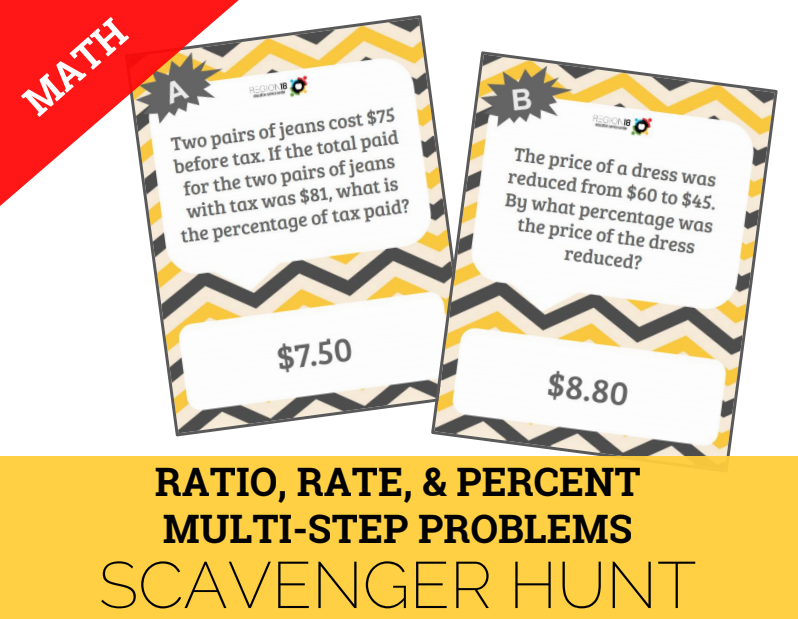Solve problems involving ratios, rates, and percents including multi-step problems involving percent increase and percent decrease, and financial literacy problems. 7.5C Similar Figures & Scale Drawings Scavenger Hunt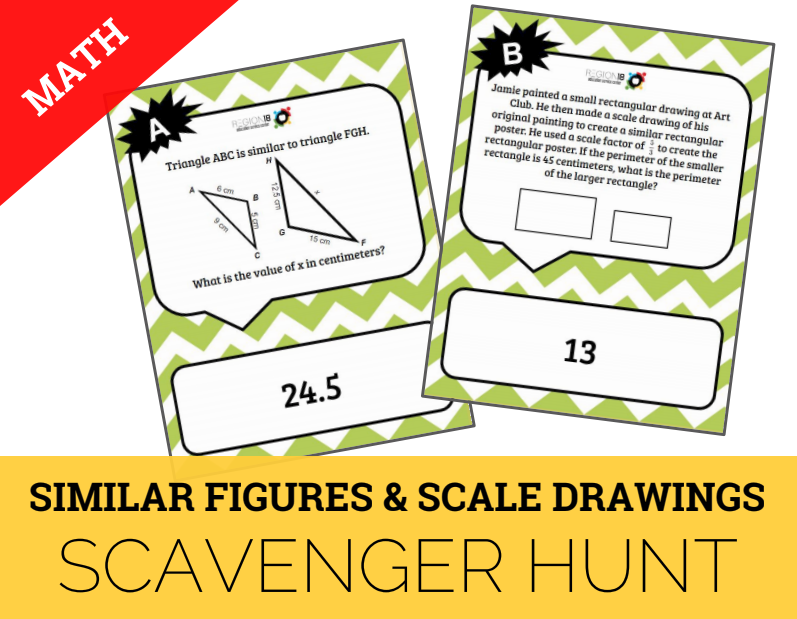Use similar shape and scale drawings to solve mathematical and real-world problems. 7.6G Bar Graph, Dot Plot, & Circle Graphs Task Cards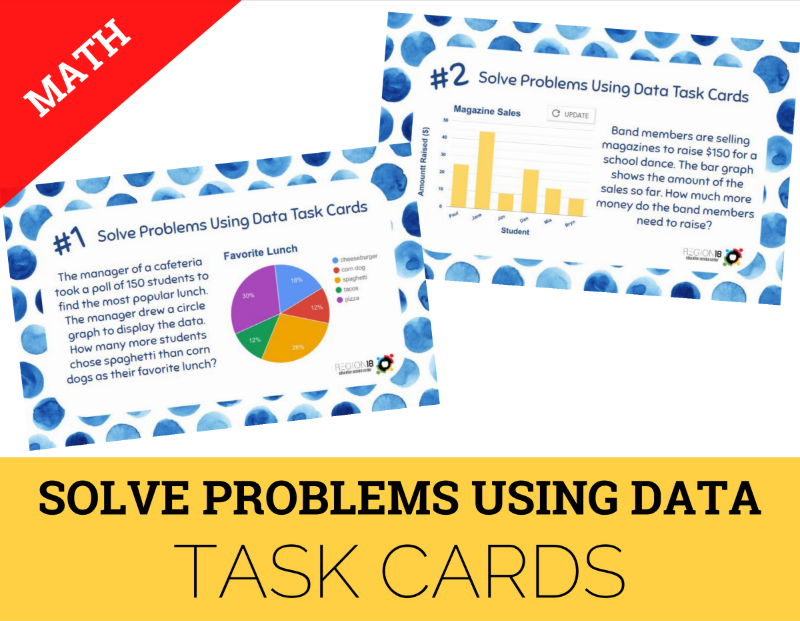Solve problems using data represented in bar graphs, dot plots, and circle graphs, including part-to-whole and part-to-part comparisons and equivalents. 7.6I Theoretical & Experimental Probability Scavenger Hunt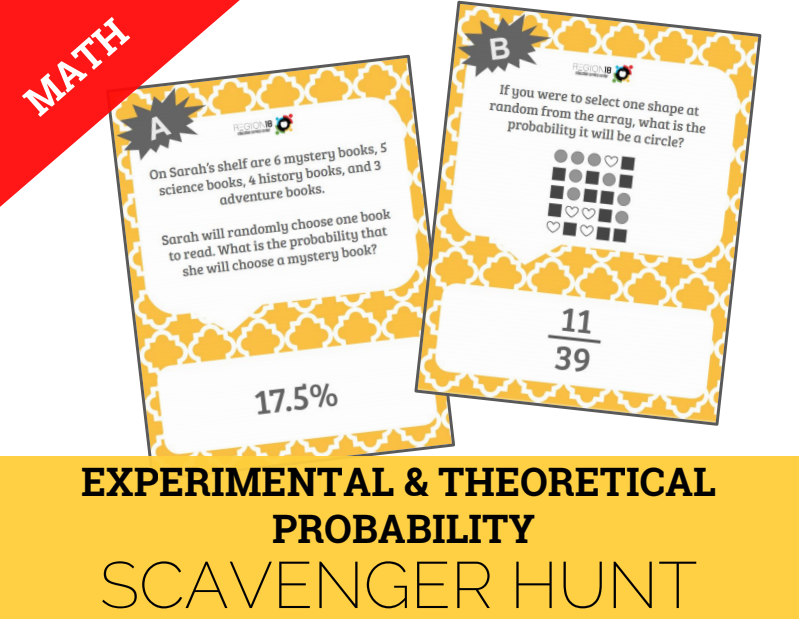Determine experimental and theoretical probabilities related to simple and compound events using data and sample spaces. 7.9A Volume of Prisms & Pyramids Sum It Up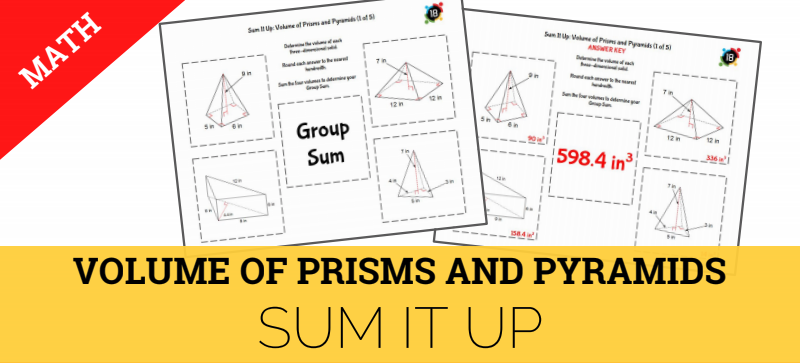Solve problems involving the volume of rectangular prisms, triangular prisms, rectangular pyramids, and triangular pyramids. 7.9B Circumference & Area of Circles Dominoes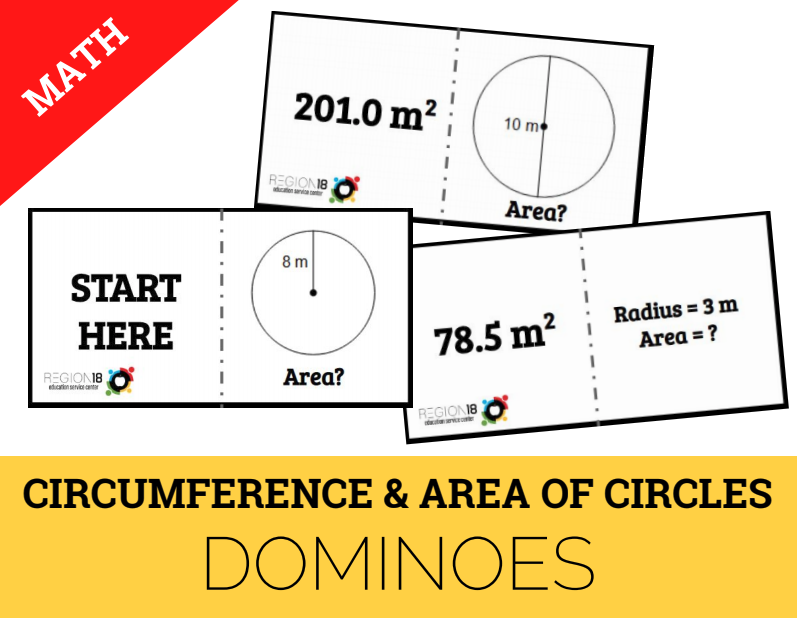Determine the circumference and area of circles. 7.9C Area of Composite Figures Pennants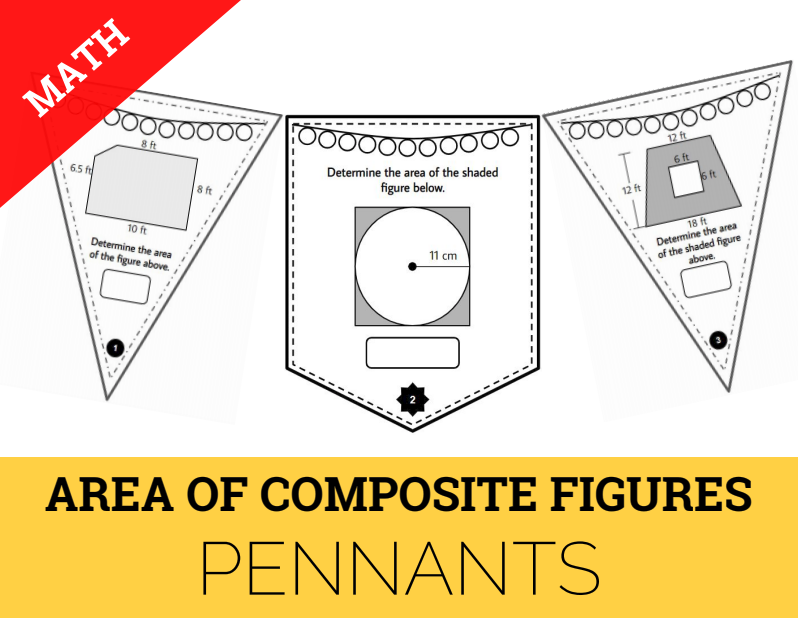Determine the area of composite figures containing combinations of rectangles, squares, parallelograms, trapezoids, triangles, semicircles, and quarter circles. 7.10B, 7.11A, 7.11B Solving Two-Step Inequalities Task Cards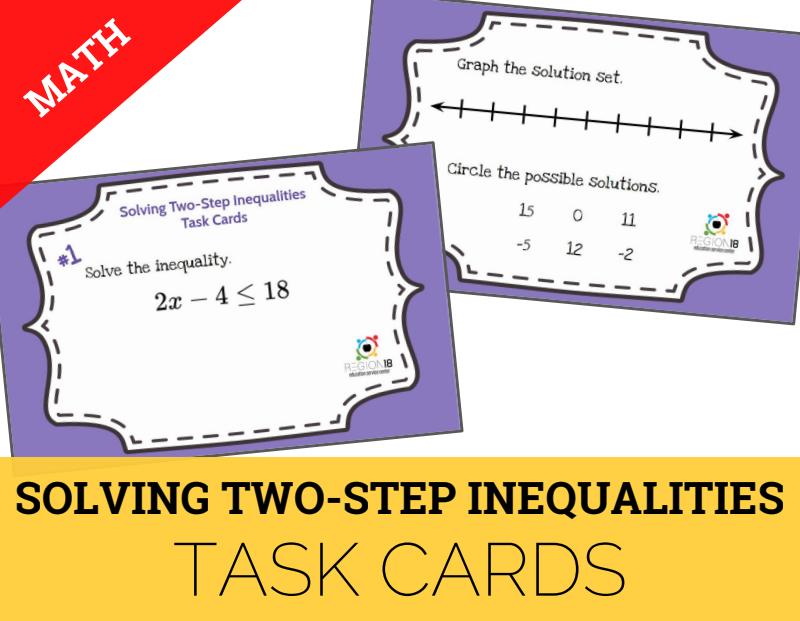Determine the area of composite figures containing combinations of rectangles, squares, parallelograms, trapezoids, triangles, semicircles, and quarter circles. 7.11A Model & Solve Two-Step Equations Model and solve two-step equations. 7.12A Comparing Box Plots & Dot Plots True/False Sort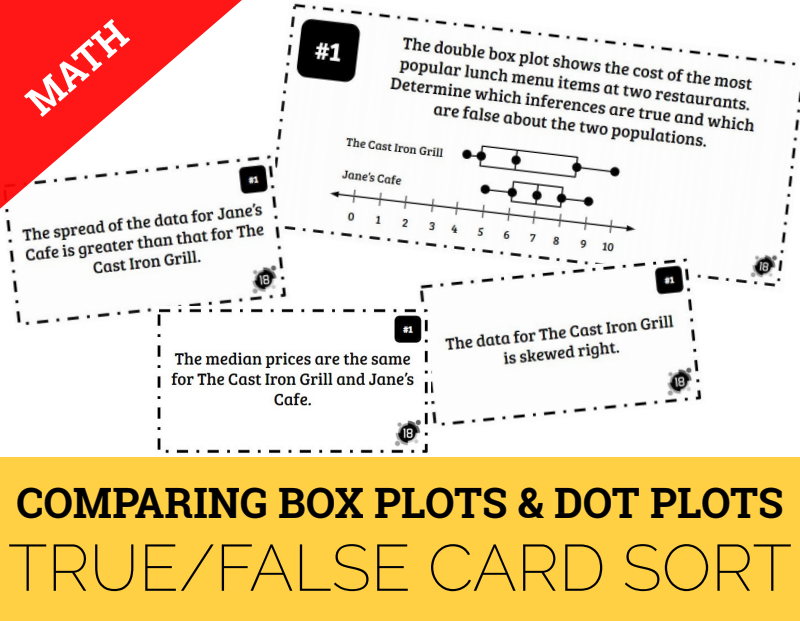Compare two groups of numeric data using comparative dot plots or box plots by comparing their shapes, centers, and spreads. Mixed Review Grade 7 Griddable Practice Task Cards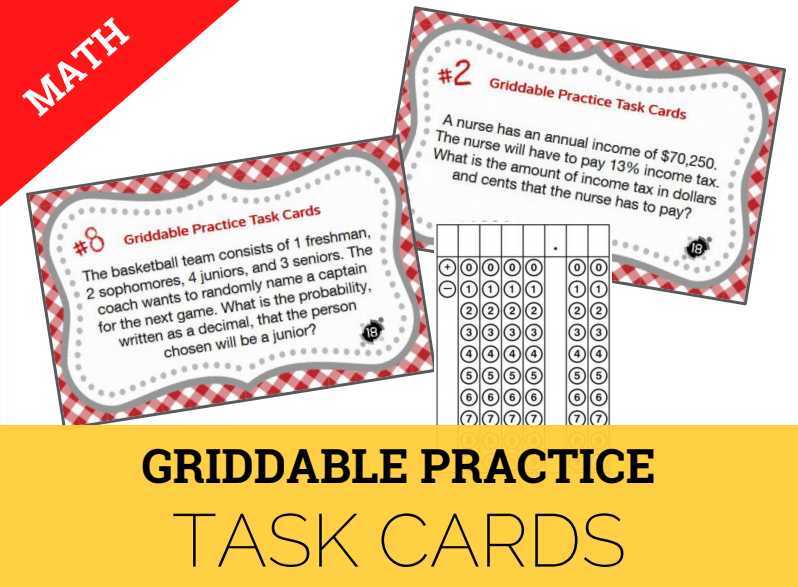Solve problems and record answers in the STAAR griddable format.